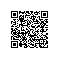# 理解 Python 中的异步编程• 批量处理程序通常是写成同步程序的：获取一些输入，处理它，然后创建一些输出。按照逻辑一步接一步地运行直到得到我们想要的输出。除了这些执行的步骤和顺序外，这类程序并不需要关注其它任何事情。

• 命令行程序通常是一个小而快的程序，用来把一些东西“转换“成其他一些东西。这个程序表现出来就是一系列程序步骤连续执行直到完成任务。

## 现实世界是异步的

• 平衡支票本是一个我们正在尝试将其完成的任务，而且我们可以把它看作一个同步任务；一步接着另一步执行直至任务完成。

• 但是，我们可以离开这个任务去洗衣服，把烘干机里已经烘干的衣物取出，再把已经洗完的衣服从洗衣机拿出来之后放到烘干机里并开始把另一些未洗的衣服放入洗衣机。不管怎样，这些任务是可以被异步完成的。

• 虽然我们在使用洗衣机和烘干机来洗衣服,这个过程是一个同步任务而且我们正在处理该任务，但是洗衣服的大部分任务是发生在我们启动洗衣机和烘干机之后发生的，这时候我们已经离开并返回平衡支票本的任务。现在任务就是异步的了，洗衣机和烘干机将会独立运行，一直到其中任意一个需要我们去处理时蜂鸣器就会响。

• 看孩子是另一个异步的任务。一旦他们起床了并且在玩耍，他们是一个人在那玩（一定程度上）直到他们需要我们的注意；一些孩子饿了，一些受伤了，一些在大声的叫喊，作为父母我们需要对此作出响应。照看孩子是一个长期运行的高优先级任务，重要性超过了任何我们可能正在做的其他任务，比如平衡支票本和洗衣服。

## 示例 1：同步编程

"""
example_1.py

Just a short example showing synchronous running of 'tasks'
"""

import queue

if work_queue.empty():
else:
while not work_queue.empty():
count = work_queue.get()
total = 0
for x in range(count):
total += 1

def main():
"""
This is the main entry point for the program
"""
# create the queue of 'work'
work_queue = queue.Queue()

# put some 'work' in the queue
for work in [15, 10, 5, 2]:
work_queue.put(work)

]

for t, n, q in tasks:
t(n, q)

if __name__ == '__main__':
main()


## 示例 2： 简单的协作并发

"""
example_2.py

Just a short example demonstrating a simple state machine in Python
"""

import queue

while not queue.empty():
count = queue.get()
total = 0
for x in range(count):
total += 1
yield

def main():
"""
This is the main entry point for the program
"""
# create the queue of 'work'
work_queue = queue.Queue()

# put some 'work' in the queue
for work in [15, 10, 5, 2]:
work_queue.put(work)

]

done = False
while not done:
try:
next(t)
except StopIteration:
done = True

if __name__ == '__main__':
main()


## 示例 3：通过阻塞调用来协作并发

"""
example_3.py

Just a short example demonstraing a simple state machine in Python
However, this one has delays that affect it
"""

import time
import queue
from lib.elapsed_time import ET

while not queue.empty():
count = queue.get()
total = 0
et = ET()
for x in range(count):
time.sleep(1)
total += 1
yield
print(f'Task {name} total elapsed time: {et():.1f}')

def main():
"""
This is the main entry point for the program
"""
# create the queue of 'work'
work_queue = queue.Queue()

# put some 'work' in the queue
for work in [15, 10, 5, 2]:
work_queue.put(work)

]
# run the scheduler to run the tasks
et = ET()
done = False
while not done:
try:
next(t)
except StopIteration:
done = True

print()
print('Total elapsed time: {}'.format(et()))

if __name__ == '__main__':
main()


## 示例 4：使用非阻塞调用来协作并发

"""
example_4.py

Just a short example demonstrating a simple state machine in Python
However, this one has delays that affect it
"""

import gevent
from gevent import monkey
monkey.patch_all()

import time
import queue
from lib.elapsed_time import ET

while not work_queue.empty():
count = work_queue.get()
total = 0
et = ET()
for x in range(count):
time.sleep(1)
total += 1
print(f'Task {name} total elapsed time: {et():.1f}')

def main():
"""
This is the main entry point for the programWhen
"""
# create the queue of 'work'
work_queue = queue.Queue()

# put some 'work' in the queue
for work in [15, 10, 5, 2]:
work_queue.put(work)

et = ET()
]
print()
print(f'Total elapsed time: {et():.1f}')

if __name__ == '__main__':
main()


## 示例 5：异步（阻塞）HTTP 下载

"""
example_5.py

Just a short example demonstrating a simple state machine in Python
URL's it gets from a queue
"""

import queue
import requests
from lib.elapsed_time import ET

while not work_queue.empty():
url = work_queue.get()
et = ET()
requests.get(url)
print(f'Task {name} total elapsed time: {et():.1f}')
yield

def main():
"""
This is the main entry point for the program
"""
# create the queue of 'work'
work_queue = queue.Queue()

# put some 'work' in the queue
for url in [
"http://yahoo.com",
"http://shutterfly.com",
"http://mypublisher.com",
]:
work_queue.put(url)

]
# run the scheduler to run the tasks
et = ET()
done = False
while not done:
try:
next(t)
except StopIteration:
done = True

print()
print(f'Total elapsed time: {et():.1f}')

if __name__ == '__main__':
main()


## 示例 6：使用 gevent 实现异步（非阻塞）HTTP 下载

"""
example_6.py

Just a short example demonstrating a simple state machine in Python
URL's it gets from a queue. It's also using gevent to get the
URL's in an asynchronous manner.
"""

import gevent
from gevent import monkey
monkey.patch_all()

import queue
import requests
from lib.elapsed_time import ET

while not work_queue.empty():
url = work_queue.get()
et = ET()
requests.get(url)
print(f'Task {name} total elapsed time: {et():.1f}')

def main():
"""
This is the main entry point for the program
"""
# create the queue of 'work'
work_queue = queue.Queue()

# put some 'work' in the queue
for url in [
"http://yahoo.com",
"http://shutterfly.com",
"http://mypublisher.com",
]:
work_queue.put(url)

et = ET()
]
print()
print(f'Total elapsed time: {et():.1f}')

if __name__ == '__main__':
main()


## 示例 7：使用 Twisted 实现异步（非阻塞）HTTP 下载

Twisted是一个非常强大的系统，采用了和 gevent 根本上不一样的方式来创建异步程序。gevent 模块是修改其模块使它们的同步代码变成异步，Twisted 提供了它自己的函数和方法来达到同样的结果。

"""
example_7.py

Just a short example demonstrating a simple state machine in Python
URL's it gets from a work_queue. This version uses the Twisted
framework to provide the concurrency
"""

from twisted.internet import defer
from twisted.web.client import getPage

import queue
from lib.elapsed_time import ET

@defer.inlineCallbacks
try:
while not work_queue.empty():
url = work_queue.get()
et = ET()
yield getPage(url)
print(f'Task {name} total elapsed time: {et():.1f}')
except Exception as e:
print(str(e))

def main():
"""
This is the main entry point for the program
"""
# create the work_queue of 'work'
work_queue = queue.Queue()

# put some 'work' in the work_queue
for url in [
b"http://yahoo.com",
b"http://shutterfly.com",
b"http://mypublisher.com",
]:
work_queue.put(url)

et = ET()
defer.DeferredList([

# run the event loop
reactor.run()

print()
print(f'Total elapsed time: {et():.1f}')

if __name__ == '__main__':
main()


## 示例8：使用 Twisted 回调函数实现异步（非阻塞）HTTP 下载

"""
example_8.py

Just a short example demonstrating a simple state machine in Python
URL's it gets from a queue. This version uses the Twisted
framework to provide the concurrency
"""

from twisted.internet import defer
from twisted.web.client import getPage

import queue
from lib.elapsed_time import ET

def success_callback(results, name, url, et):
print(f'Task {name} total elapsed time: {et():.1f}')

if not queue.empty():
while not queue.empty():
url = queue.get()
et = ET()
d = getPage(url)
yield d

def main():
"""
This is the main entry point for the program
"""
# create the queue of 'work'
work_queue = queue.Queue()

# put some 'work' in the queue
for url in [
b"http://yahoo.com",
b"http://shutterfly.com",
b"http://mypublisher.com",
]:
work_queue.put(url)

et = ET()

# create cooperator

defer.DeferredList([

# run the event loop
reactor.run()

print()
print(f'Total elapsed time: {et():.1f}')

if __name__ == '__main__':
main()


## 结论使用钉钉扫一扫加入圈子
+ 订阅